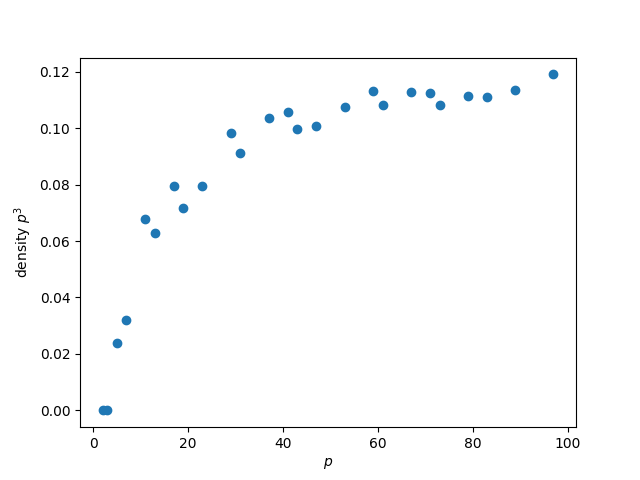# Piranhas and prime factors

The piranha problem says an event cannot be highly correlated with a large number of independent predictors. If you have a lot of strong predictors, they must predict each other, analogous to having too many piranhas in a small body of water: they start to eat each other.

The piranha problem is subtle. It can sound obvious or mysterious, depending on how you state it. You can find several precise formulations of the piranha problem here.

## Prime piranhas

An analog of the piranha problem in number theory is easier to grasp. A number N cannot have two prime factors both larger than its square root, nor can it have three prime factors all larger than its cube root. This observation is simple, obvious, and useful.

For example, if N is a three-digit number, then the smallest prime factor of N cannot be larger than 31 unless N is prime. And if N has three prime factors, at least one of these must be less than 10, which means it must be 2, 3, 5, or 7.

There are various tricks for testing divisibility by small primes. The tricks for testing divisibility by 2, 3, and 5 are well known. Tricks for testing divisibility by 7, 11, and 13 are moderately well known. Tests for divisibility by larger primes are more arcane.

Our piranha-like observation about prime factors implies that if you know ways to test divisibility by primes less than p, then you can factor all numbers up to p² and most numbers up to p³. The latter part of this statement is fuzzy, and so we’ll explore it a little further.

## How much is “most”?

For a given prime p, what proportion of numbers less than p³ have two factors larger than p? We can find out with the following Python code.

```    from sympy import factorint

def density(p, N = None):
if not N:
N = p**3
count = 0
for n in range(1, N):
factors = factorint(n).keys()
if len([k for k in factors if k > p]) == 2:
count += 1
return count/N
```

The code is a little more general than necessary because in a moment we will like to consider a range that doesn’t necessarily end at p³.

Let’s plot the function above for the primes less than 100.Short answer: “most” means roughly 90% for primes between 20 and 100.

The results are very similar if we pass in a value of N greater than p³. About 9% of numbers less than 1,000 have two prime factors greater than 10, and about 12% of numbers less than 1,000,000 have two prime factors greater than 100.